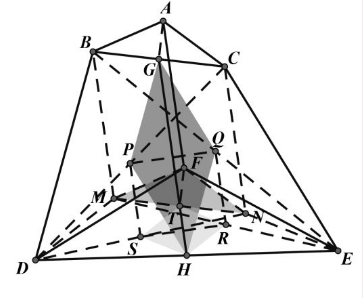【答案】

$Q 、 R 、 S$ 分别是 $C D 、 B E 、 E M 、 D N$ 中点, 则四边形
$A B M F 、 A C N F 、 A G T F 、 B C N M$ 都是平行四边形。

$$P H \boxminus \frac{1}{2} C E \boxminus G Q, \quad P G \boxminus \frac{1}{2} B D \square H Q$$

$$P S \equiv \frac{1}{2} C N \equiv \frac{1}{2} B M \boxminus Q R, \quad \therefore P Q / / S R,$$
$\therefore T$ 在 $G H$ 上, 又 $G T / / A F$
$\therefore A F / / G H$ 。